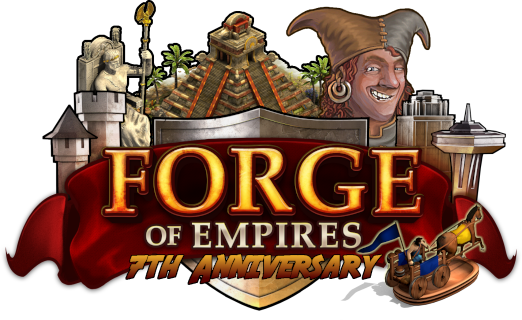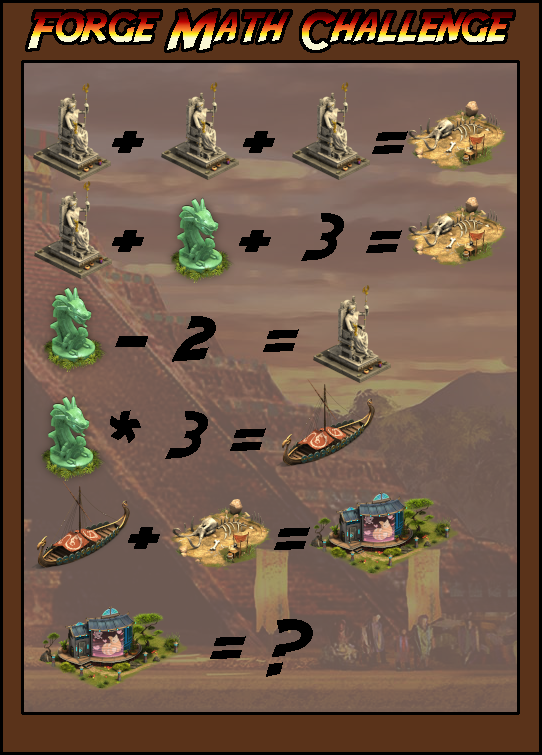# Contest day 6

Status
Not open for further replies.

#### DeletedUser39714Greetings, Queens and Kings!

2018 brought the Reconstruction Mode to the game, which was one of the most requested features of the game. The research "Mathematics" was needed to unlock it, but now it is on you to use some mathematics to solve the following puzzle!For this contest, 7 players can win 250 goods of your choice! Have fun calculating!

Please submit the correct answer until April 28, 2019. We will pick the 7 lucky winners from all correct answers!Sincerely,

I believe these are the possible answers to the equation:
a) 5 jade statues + 1 = 36
b) 3 jade statues + 3 zeus statues = 21 + 15 = 36
c) 3 jade statues + 2 zeus + 5 = 21 + 10 + 5 =36
d) 3 jade statues + 15 = 21 + 15 = 36
e) 21 +15 = 36

The zeus statue = 5
The boat = 21
The bones = 15

Last edited by a moderator:

36

5+5+5=15
5+7+3=15
7-2=5
7*3=21
21+15=36

36

#### Scalius the Just

##### Member
a + a + a = b
a + c + 3 = b
c - 2 = a
c * 3 = d
d + b = e
e = ?

a + (a + 2) + 3 = 3a
2a + 5 = 3a
a = 5
c = 7
b = 15
d = 21
e = 36

36

#### DeletedUser39604

36.

3z=Bones
3z=15
(Z)eus=5
Z + r +3 = Bones
5+r+ 3=15
(R) relic = 7
(F) fishing hut= boat * Bones
(F)= r*3 + 3z
(F)= 21 + 15
(F) = 36

36

5+5=5=15
5+7+3=15
7-2=5
7*3=21
21+15=36

#### DeletedUser39096

Answer to contest 6 is 36

#### DeletedUser31427

36. Maggie-May (Xyr)

#### DeletedUser39637Greetings, Queens and Kings!

2018 brought the Reconstruction Mode to the game, which was one of the most requested features of the game. The research "Mathematics" was needed to unlock it, but now it is on you to use some mathematics to solve the following puzzle!For this contest, 7 players can win 250 goods of your choice! Have fun calculating!

Please submit the correct answer until April 28, 2019. We will pick the 7 lucky winners from all correct answers!Sincerely,

Should be 36
kind regards,
Bagheera1

36

#### DeletedUser39897

Steelth says answer is 36. (Zeus = 5, relic = 7, boat = 21, excavation site = 15)

36

36

#### qaccy

36

##### Member
3 Green Dragons and 3 Neptunes Thrones.

#### DeletedUser38879

Zeus = 5
Bones= 15
Relic = 7
Boat = 21
House = 36

Zeus(5)+Zeus(5)+Zeus(5)=Bones(15)
Zeus(5)+Relic(7)+(3)=Bones(15)
Relic(7)-(2)=Zeus(5)
Relic(7)*(3)=Boat(21)
Boat(21)+Bones(15)=House(36)

House=36

Status
Not open for further replies.